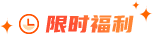### 寻找两个正序数组的中位数题目描述

给定两个大小为 m 和 n 的正序(从小到大)数组 nums1 和 nums2. 请你找出这两个正序数组的中位数，并且要求算法的时间复杂度为 O(log(m + n))。 你可以假设 nums1 和 nums2 不会同时为空。

示例1：

nums1 = [1. 3]

nums2 = 

则中位数是 2.0

示例2：

nums1 = [1. 2]

nums2 = [3. 4]

则中位数是 (2 + 3)/2 = 2.5

题目解析

这道题网络上的解析都非常“高深”，很难理解。私以为它们都将简单的问题复杂化了。本题在一些处理上确实会有些麻烦，比如数组边界的处理，和偶数个数的中位数的处理。但其核心思想并不复杂。

首先，我们可以只考虑数字总个数为奇数的情况。让我们看下下图：

蓝框是中位数左边的数(包括中位数)，而橘框则为中位数右边的数。

3个显然的规则： 1.两个数组的蓝框总个数=(数字总个数+1)/2; 2.所有蓝框内的数都小于橘框内的数 3.中位数为蓝框中最大的那一位(即数组1蓝框最后一位，或数组2蓝框最后一位)

如图，我们要找到一组A，B，满足上面3条规则。 对于规则1.我们在数组1中找任意A，然后根据规则1就能推算出对应的B的位置。 对于规则2.由于数组1和2都是有序数组，即X1

那么具体该如何操作呢? 由于数组1和2都是有序数组，且题目要求O(log(m+n))复杂度，我们明显应考虑二分法。

情况1：

首先，我们选择数组1进行操作。取其中间值9 。(因此 A=9) 根据规则1.我们在数组2中找到对应值(B = 4)。(一共有11个数，(11+1) / 2 = 6.因此蓝色框总数为6) 紧接着，我们根据规则2判断A(9)是否小于B.next(5)，以及B(4)是否小于A.next(11)。 显然，A比B.next,也就是一个橘框还要大。这是不允许的。可见A只能取比9更小的数字了。如果取更大的数字，那B就会更小，更不可能满足规则2.所以这种情况下我们要向左进行二分。

情况2：

这种情况下B比A.next,也就是一个橘框还要大。这是不允许的。可见A只能取比9更大的数字了。如果取更小的数字，那B就会更大，更不可能满足规则2.所以这种情况下我们要向右进行二分。

情况3：

随着我们不断地二分，中位数显然必然会出现。 如图上这种情况，A小于B.next，且B小于A.next。 那么，显然，A,B中较大的那一项就是中位数(规则3)。

本题算法的核心思想就是这样简单。此外，当数字总数为偶数时，我们需要把我们求得的“中位数"与它下一项相加并除以2即可。由于本题中数字可能相同，所以大小的比较需要使用>=和<=。 下面提供了作者的一份代码，leetcode上的结果为：执行用时：2 ms;内存消耗：40.3 MB，都超过了100%的用户。读者可以参考一下。

代码实现

Java语言

public class Solution {

public double findMedianSortedArrays(int[] nums1. int[] nums2) {

// 使nums1成为较短数组,不仅可以提高检索速度,同时可以避免一些边界问题

if (nums1.length > nums2.length) {

int[] temp = nums1;

nums1 = nums2;

nums2 = temp;

}

int len1 = nums1.length;

int len2 = nums2.length;

int leftLen = (len1 + len2 + 1) / 2; //两数组合并&排序后,左半边的长度

// 对数组1进行二分检索

int start = 0;

int end = len1;

while (start <= end) {

// 两个数组的被测数A,B的位置(从1开始计算)

// count1 = 2 表示 num1 数组的第2个数字

// 比index大1

int count1 = start + ((end - start) / 2);

int count2 = leftLen - count1;

if (count1 > 0 && nums1[count1 - 1] > nums2[count2]) {

// A比B的next还要大

end = count1 - 1;

} else if (count1 < len1 && nums2[count2 - 1] > nums1[count1]) {

// B比A的next还要大

start = count1 + 1;

} else {

// 获取中位数

int result = (count1 == 0)? nums2[count2 - 1]: // 当num1数组的数都在总数组右边

(count2 == 0)? nums1[count1 - 1]: // 当num2数组的数都在总数组右边

Math.max(nums1[count1 - 1], nums2[count2 - 1]); // 比较A,B

if (isOdd(len1 + len2)) {

return result;

}

// 处理偶数个数的情况

int nextValue = (count1 == len1) ? nums2[count2]:

(count2 == len2) ? nums1[count1]:

Math.min(nums1[count1], nums2[count2]);

return (result + nextValue) / 2.0;

}

}

return Integer.MIN_VALUE; // 绝对到不了这里

}

// 奇数返回true,偶数返回false

private boolean isOdd(int x) {

return (x & 1) == 1;

}

}### 大数据师资团队更多>>

#### 开班信息 北京校区 北京校区 大连校区 广州校区 成都校区 杭州校区 长沙校区 合肥校区 南京校区 上海校区 深圳校区 武汉校区 郑州校区 西安校区 青岛校区 重庆校区 太原校区 沈阳校区